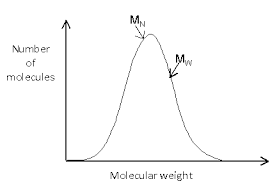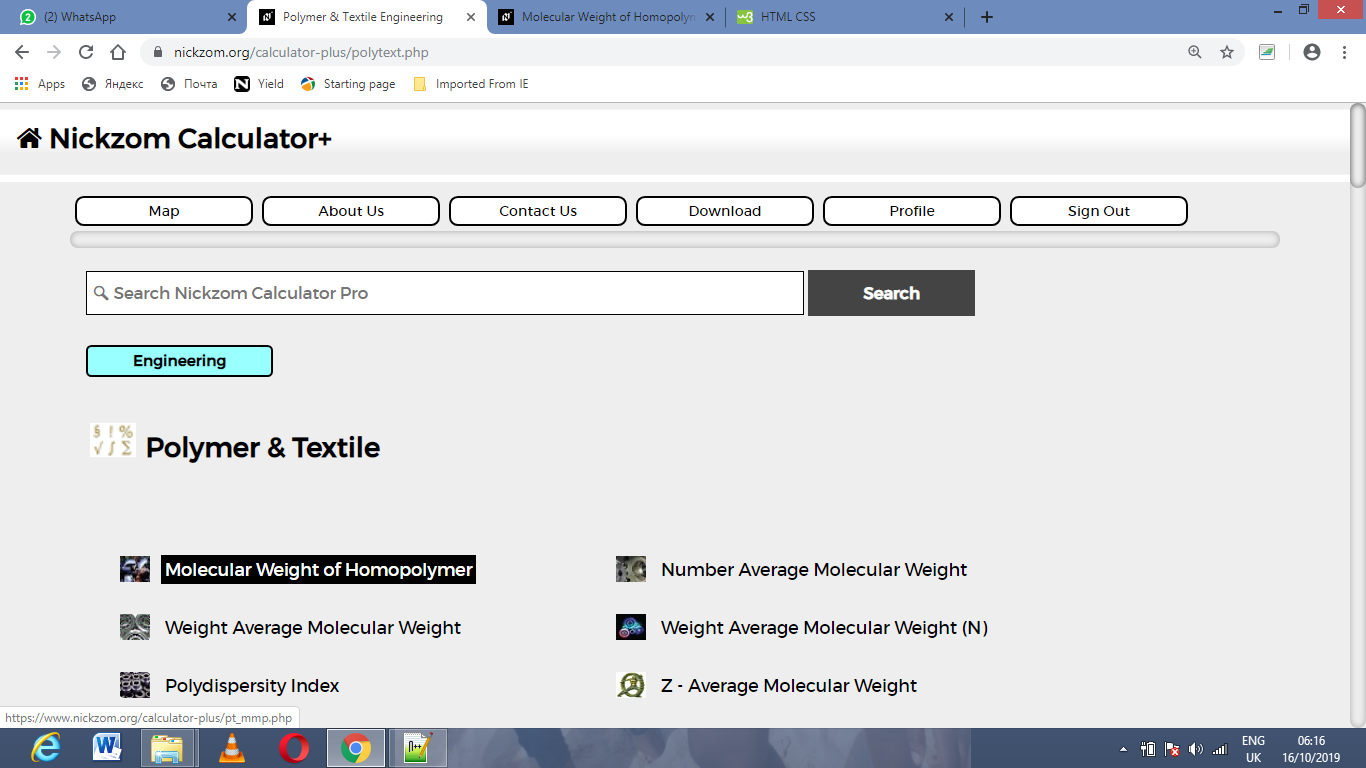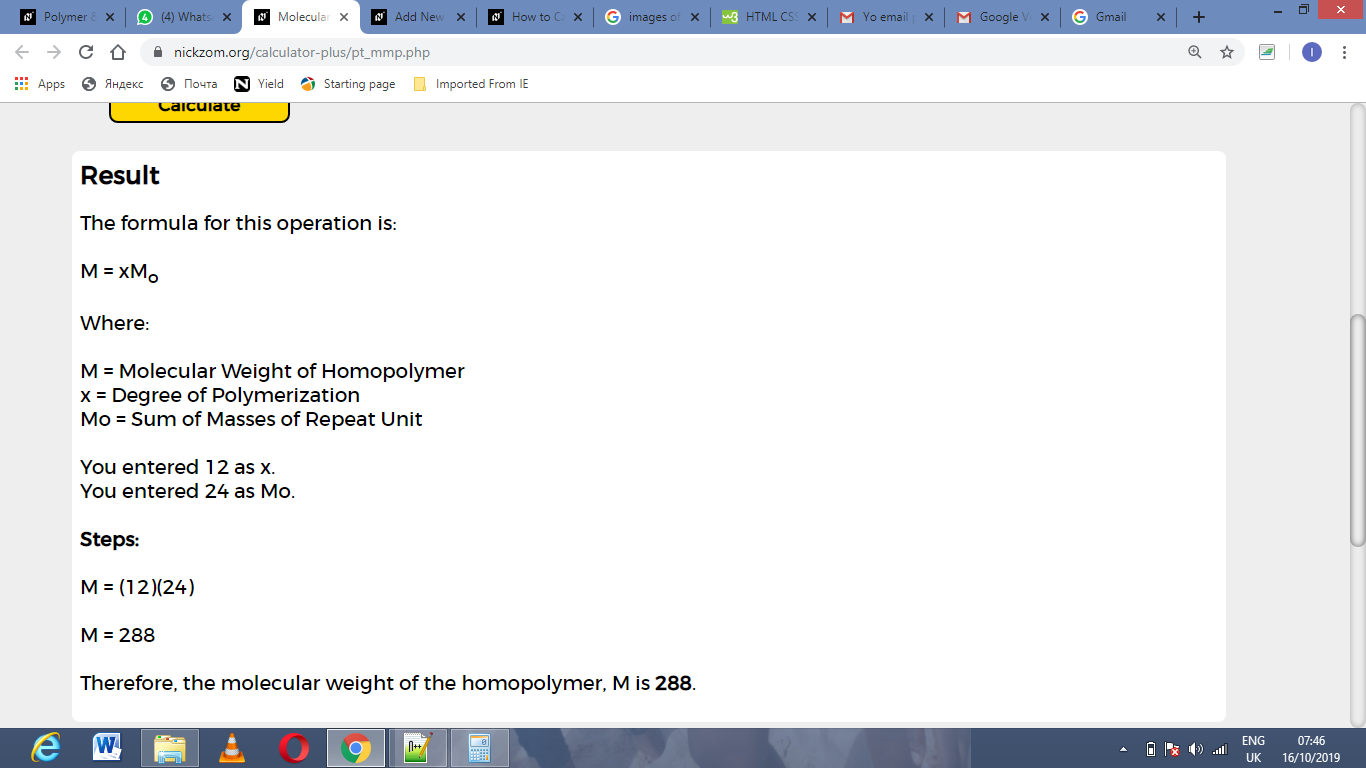# How to Calculate and Solve for Molecular Weight of Homopolymer | Polymer & TextileThe image above represents the molecular weight of homopolymer.

To compute for molecular weight of homopolymer, two essential parameters are needed and these parameters are Degree of Polymerization (x) and Sum of Masses of Repeat Unit (Mo).

The formula for calculating the molecular weight of homopolymer:

M = xMo

Where;

M = Molecular Weight of Homopolymer
x = Degree of Polymerization
Mo = Sum of Masses of Repeat Unit

Let’s solve an example;
Given that the degree of polymerization is 12 and the sum of masses of repeat unit is 24. Find the molecular weight of homopolymer?

This implies that;

x = Degree of Polymerization = 12
Mo = Sum of Masses of Repeat Unit = 24

M = xMo
M = (12)(24)
M = 288

Therefore, the molecular weight of the homopolymer is 288.

Calculating the Degree of Polymerization when the Molecular Weight of Homopolymer and the Sum of Masses of Repeat Unit is Given.

x = M / Mo

Where;

x = Degree of Polymerization
M = Molecular Weight of Homopolymer
Mo = Sum of Masses of Repeat Unit

Let’s solve an example;
Given that the molecular weight of homopolymer is 140 and the sum of masses of repeat unit is 70. Find the degree of polymerization?

This implies that;

M = Molecular Weight of Homopolymer = 140
Mo = Sum of Masses of Repeat Unit = 70

x = M / Mo
x = 140 / 70
x = 2

Therefore, the degree of polymerization is 2.

Calculating the Sum of Masses of Repeat Unit when the Molecular Weight of Homopolymer and the Degree of Polymerization is Given.

Mo = M / x

Where;

Mo = Sum of Masses of Repeat Unit
M = Molecular Weight of Homopolymer
x = Degree of Polymerization

Let’s solve an example;
Given that the degree of polymerization is 14 and the molecular weight of homopolymer is 280. Find the sum of masses of repeat unit?

This implies that;

M = Molecular Weight of Homopolymer = 280
x = Degree of Polymerization = 14

Mo = M / x
Mo = 280 / 14
Mo = 20

Therefore, the sum of masses of repeat unit is 20.

Nickzom Calculator – The Calculator Encyclopedia is capable of calculating the molecular weight of homopolymer.

To get the answer and workings of the molecular weight of homopolymer using the Nickzom Calculator – The Calculator Encyclopedia. First, you need to obtain the app.

You can get this app via any of these means:

To get access to the professional version via web, you need to register and subscribe for NGN 1,500 per annum to have utter access to all functionalities.
You can also try the demo version via https://www.nickzom.org/calculator

Apple (Paid) – https://itunes.apple.com/us/app/nickzom-calculator/id1331162702?mt=8
Once, you have obtained the calculator encyclopedia app, proceed to the Calculator Map, then click on Polymer & Textile under Engineering.Now, Click on Molecular Weight of Homopolymer under Polymer & TextileThe screenshot below displays the page or activity to enter your values, to get the answer for the molecular weight of homopolymer according to the respective parameters which are the Degree of Polymerization (x) and Sum of Masses of Repeat Unit (Mo).Now, enter the values appropriately and accordingly for the parameters as required by the Degree of Polymerization (x) is 12 and Sum of Masses of Repeat Unit (Mo) is 24.Finally, Click on CalculateAs you can see from the screenshot above, Nickzom Calculator– The Calculator Encyclopedia solves for the molecular weight of homopolymer and presents the formula, workings and steps too.